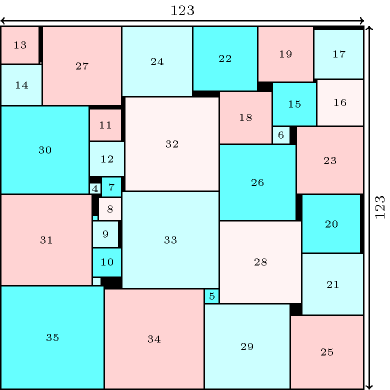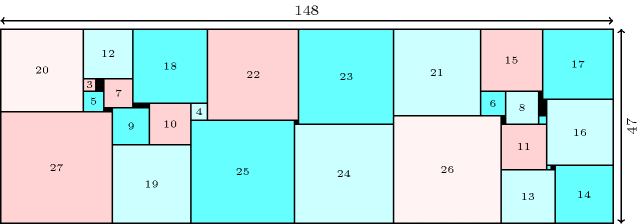### 3.7.235. Smallest rectangle area

Denotes that a constraint can be used for finding the smallest rectangle area where one can pack a given set of rectangles (or squares). A first example of such packing problem attributed to S. W. Golomb is to find the smallest square that can contain the set of consecutive squares from $1×1$ up to $n×n$ so that these squares do not overlap each other. A program using the $\mathrm{𝚍𝚒𝚏𝚏𝚗}$ constraint was used to construct such a table for $n\in \left\{1,2,\cdots ,25,27,29,30\right\}$ in [BeldiceanuBourreauChanRivreau97]. New optimal solutions for this problem were found in [SimonisSullivan08] for $n=26,31,35$. Figure 3.7.66 gives the solution found for $n=35$ by H. Simonis and B. O'Sullivan. Algorithms and lower bounds for solving the same problem can also be respectively found in

##### Figure 3.7.66. Smallest square (of size 123) for packing squares of size $1,2,\cdots ,35$In his paper (i.e., [Korf04]), Richard E. Korf also considers the problem of finding the minimum-area rectangle that can contain the set of consecutive squares from $1×1$ up to $n×n$ and solve it up to $n=25$. In 2008 this value was improved up to $n=27$ by H. Simonis and B. O'Sullivan [SimonisSullivan08]. Figure 3.7.67 gives the solution found for $n=27$ by H. Simonis and B. O'Sullivan.

##### Figure 3.7.67. Rectangle with the smallest surface (of size $148×47$) for packing squares of size $1,2,\cdots ,27$